# 【Java基础 二】---面向对象思想（基础）

##基本概念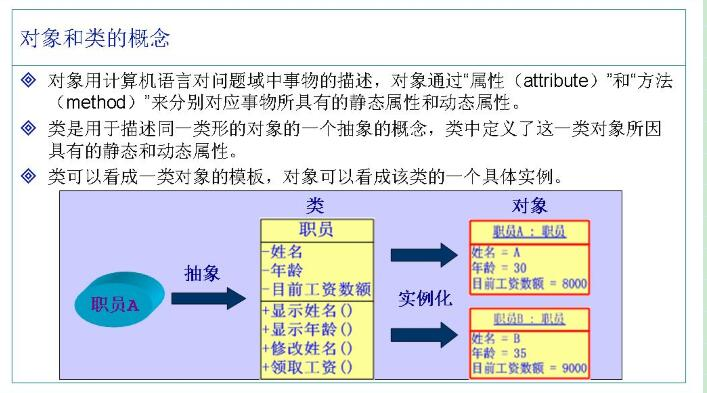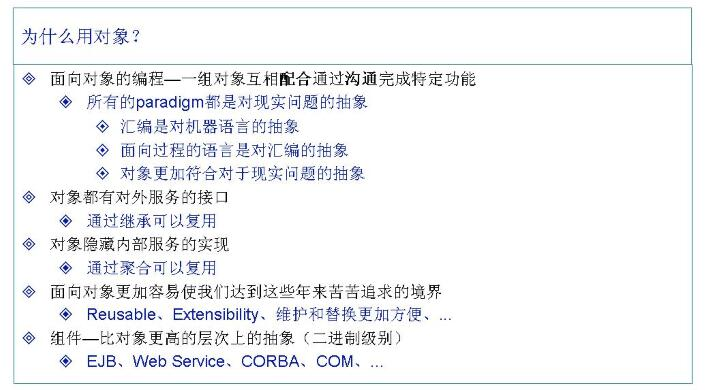##对象之间的关系

###关联关系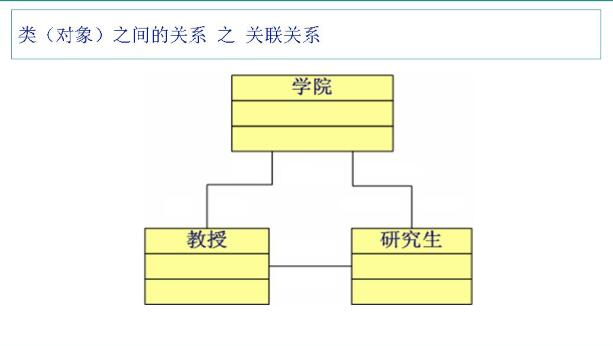###继承关系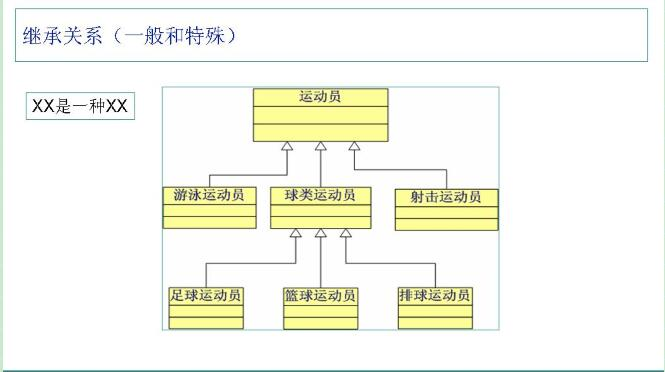###聚合关系（松耦合）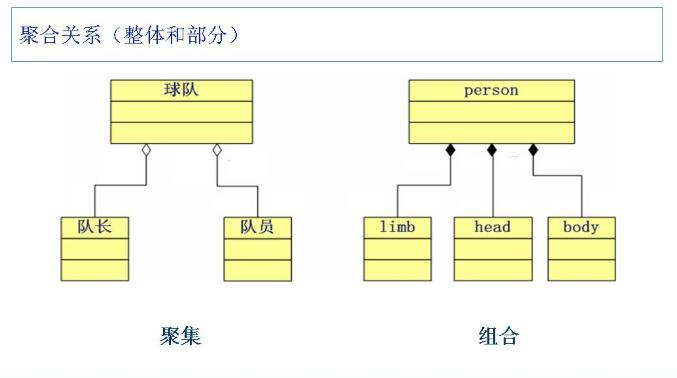###继承关系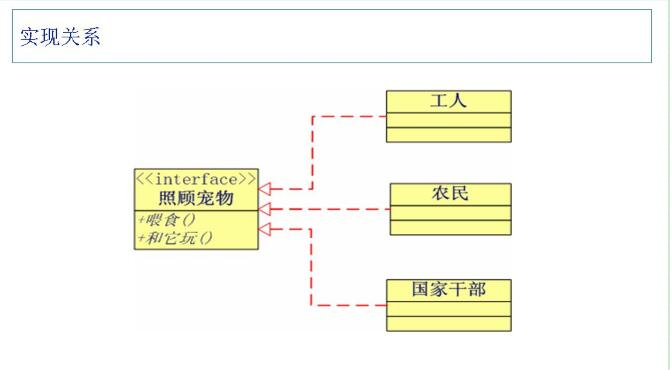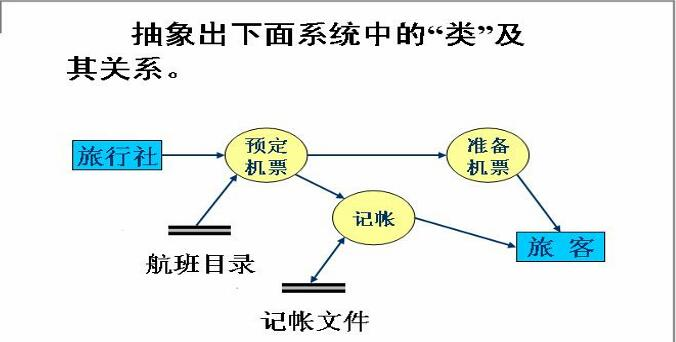1：具有的类：旅行社，机票，旅客，账户，目录
2：机票：时间，价格，班次，（作废，显示价格，显示航班时间）。旅行社：（预定，记录，准备）。航班目录：航班号，时间，地点。账户：金额，总额，（收入，支出）。旅客：姓名，年龄，性别（预定）
3：旅客从旅行社预定机票，旅行社查看航班目录，预定机票，记录到账户，把机票准备好

1：人，车，新疆
2：人：（驾驶）。车：颜色，大小，型号，油门，刹车（踩油门，踩刹车）。新疆：大小。
3：人开着车去到新疆。
##代码举例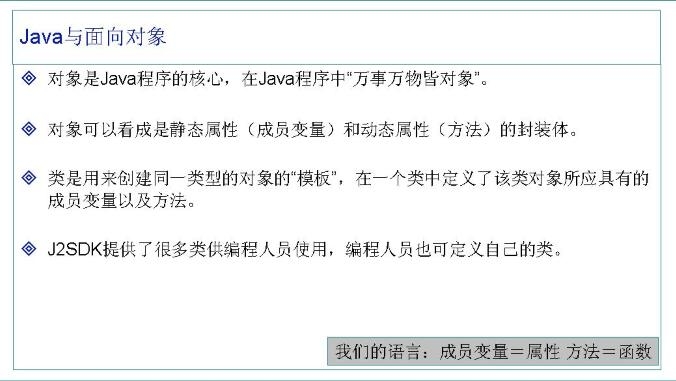public class Dog {                                 //类名首字母大写
public String furColor;                       //运用驼峰标识
public String height;
public String weight;                         //变量名方法名首字母都是小写

public void catchMouse(mouse m){    //建立狗和老鼠之间的关联关系
m.scream();
}

}
+++++++++++++++++++++++++++++++++++++++++++++++++++++++++++++++++++++++
public class mouse {
void scream(){
System.out.println("狗拿耗子多管闲事");
}
}
+++++++++++++++++++++++++++++++++++++++++++++++++++++++++++++++++++++++
public class test {
public static void main(String[] args) {
Dog dog=new Dog();
mouse m=new mouse();      //测试类里要把所有类实例化为对象，然后把握对象之间的关系
dog.catchMouse(m);
}

}



##构造方法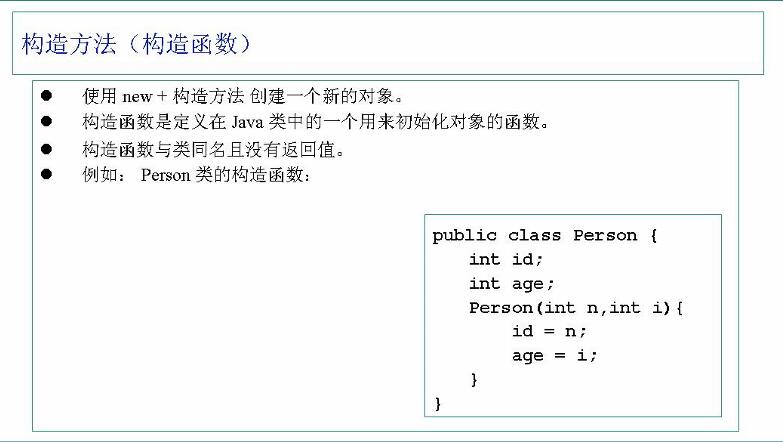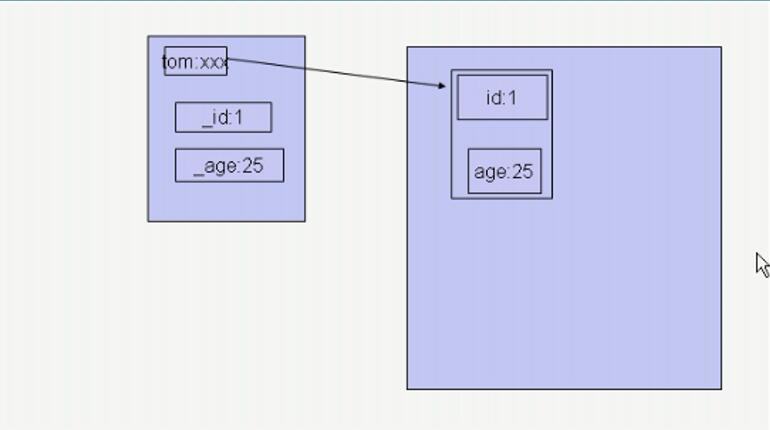##方法调用过程（重要）

class BirthDate {
private int day;
private int month;
private int year;

public BirthDate(int d, int m, int y) {                 //构造方法
day = d;
month = m;
year = y;
}

public void setDay(int d) {
day = d;
}

public void setMonth(int m) {
month = m;
}

public void setYear(int y) {
year = y;
}

public int getDay() {
return day;
}

public int getMonth() {
return month;
}

public int getYear() {
return year;
}                                   //设置各项值

public void display() {
System.out.println
(day + " - " + month + " - " + year);
}
}

public class Test{
public static void main(String args[]){
Test test = new Test();             //在堆里new出一个Test
int date = 9;                        //局部变量date为9
BirthDate d1= new BirthDate(7,7,1970);
BirthDate d2= new BirthDate(1,1,2000);
test.change1(date);                //把date的值赋给i，然后令i=1234，接下来栈里的i消失，date任然在
test.change2(d1);                  //把birthdate类型的d1赋给b，然后new出一块儿新的内存，该新内存并没有改变原来内存的值，等待垃圾回收，同样d1消失
test.change3(d2);                  //通过setday方法成功改值，同样d2消失
System.out.println("date=" + date);
d1.display();
d2.display();
}

public void change1(int i){
i = 1234;
}

public void change2(BirthDate b) {
b = new BirthDate(22,2,2004);
}

public void change3(BirthDate b) {
b.setDay(22);
}
}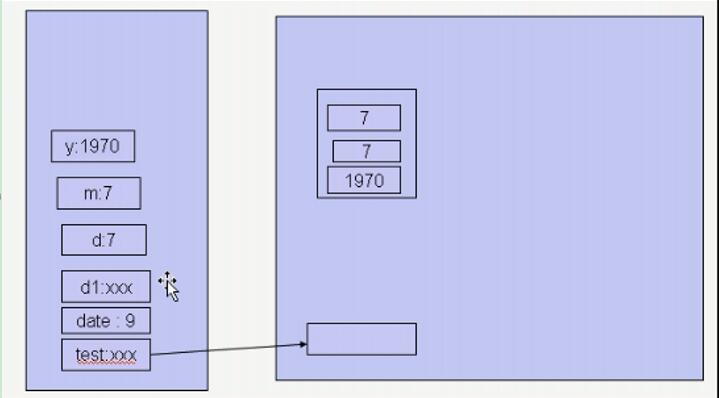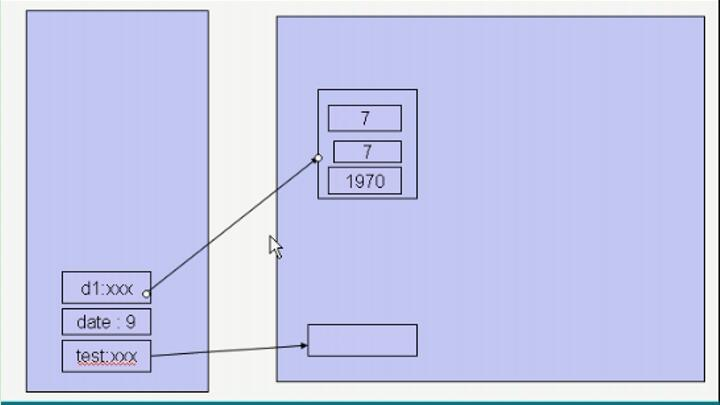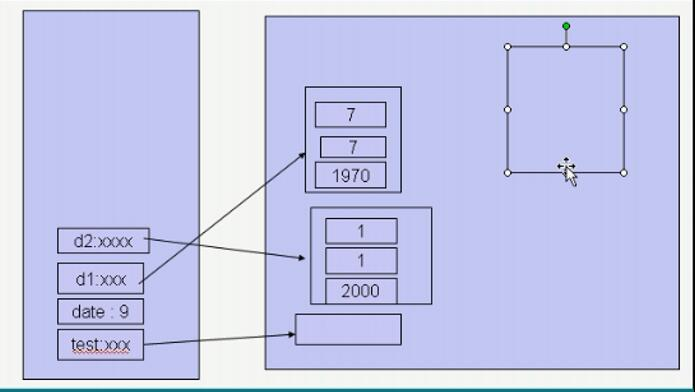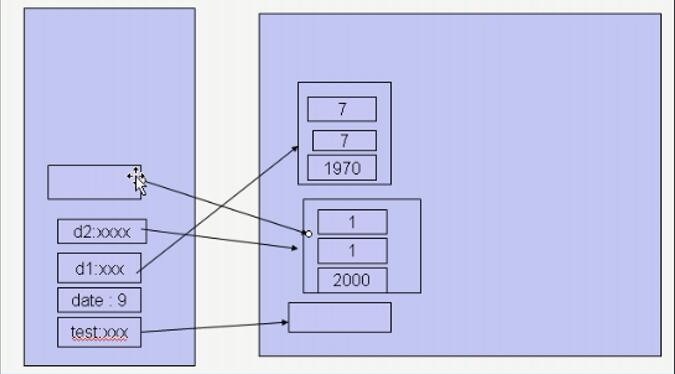###内存分析举例

public class Point {
double x;
double y;
double z;

public Point(double x, double y, double z) {
this.x = x;
this.y = y;
this.z = z;
}

public void setX(double x) {
this.x = x;
}

public void setY(double y) {
this.y = y;
}

public void setZ(double z) {
this.z = z;
}

public void distance(Point p){                 //计算两点之间的距离
double dis=0;
dis= Math.sqrt((x-p.x)*(x-p.x)+(y-p.y)*(y-p.y)+(z-p.z)*(z-p.z));
System.out.println(dis);

}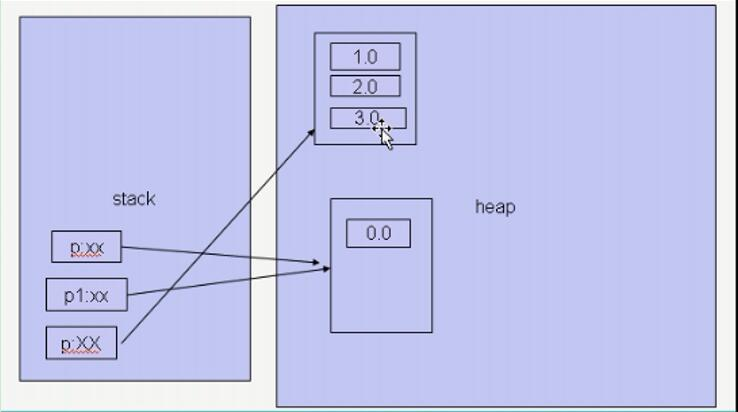public class Testpoint {

public static void main(String[] args) {
Point p1 = new Point(1, 1, 1);    //新建引用数据类型，在棧里和堆里都有内存空间占用
System.out.println(p1.x);
Point p = new Point(1, 1, 1);
System.out.println(p.y);
System.out.println(p.z);
p.setX(2);
p.setY(2);
p.setZ(2);
System.out.println(p.x);
System.out.println(p.y);
System.out.println(p.z);
p.distance(p1);           //当调用方法的时候，在栈里出现一个形式参数p也指p1指向的对象，计v算结束后得到返回值并打印出来，然后p与返回值都消失。
p.distance(new Point(2, 2, 2));   //当调用方法的时候在堆里new出一块新空间，成员变量在堆里分配（该新空间的赋值也是三个形式参数完成的）但是并没有任何引用指向它，所以被形式参数指向，计算结束后，棧里的p消失，三个形参位，x，y，z也都消失。                                                                                                                                                                                                                                                                                                                                                                                                                                                                                                                                                                                                                                                                                                                                                                                                                                                                                                                                                                                                                                                                                                                                                                                                                                                                                                                                                                                                                                                                                                                                                                                                                                                                                                                                                                                                                                                                                                                                                                                                                                                                                                                                                                                                                                                                                                                                                                                                                                                                                                                                                                                                                                                                                                                                                                                                                                                                                                                                                                                                                                                                                                                                                                                                                                                                                                                                                                                                                                                                                                                                                                                                                                                                                                                                                                                                                                                                                                                                                                                                                                                                                                                                                                                                                                                                                                                                                                                                                                                                                                                                                                                                                                                                                                                                                                                                                                                                                                                                                                                                                                                                                                                                                                                                                                                                                                                                                                                                                                                                                                                                                                                                                                                                                                                                                                                                                                                                                                                                                                                                                                                                                                                                                                                                                                                                                                                                                                                                                                                                                                                                                                                                                                                                                                                                                                                                                                                                                                                                                                                                                                                                                                                                                                                                                                                                                                                                                                                                                                                                                                                                                                                                                                                                                                                                                                                                                                                                                                                                                                                                                                                                                                                                                                                                                                                                                                                                                                                                                                                                                                                                                                                                                                                                                                                                                                                                                                                                                                                                                                                                                                                                                                                                                                                                                                                                                                                                                                                                                                                                                                                                                                                                                                                                                                                                                                                                                                                                                                                                                                                                                                                                                                                                                                                                                                                                                                                                                                                                                                                                                                                                                                                                                                                                                                                                                                                                                                                                                                                                                                                                                                                                                                                                                                                                                                                                                                                                                                                                                                                                                                                                                                                                                                                                                                                                                                                                                                                                                                                                                                                                                                                                                                                                                                                                                               堆里的那块儿内存由于没有任何对象指向它，所以被垃圾收集器回收
}



##方法重载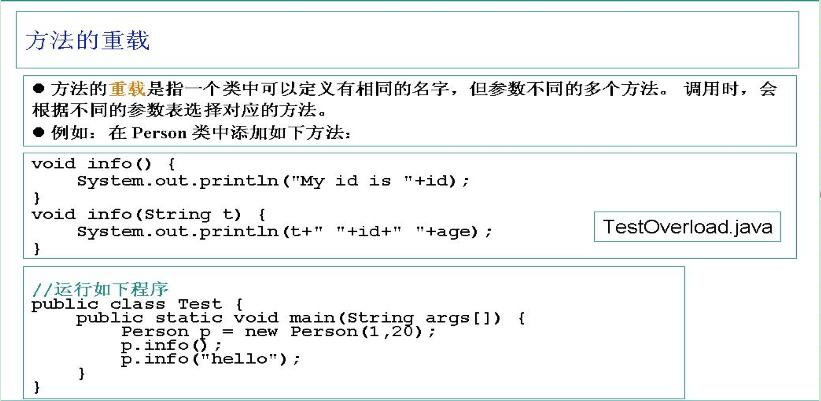**名字相同，参数不同：**参数个数不一样或者是参数类型不一样，只要有一种不一样就构成重载。返回值类型不同不能够作为重载的理由
###内存分析举例

public class Point {
public double x;
public double y;

public double getX() {
return x;
}

public void setX(double x) {
this.x = x;
}

public double getY() {
return y;
}

public void setY(double y) {
this.y = y;
}

public Point(double x, double y) {
this.x = x;
this.y = y;
}
+++++++++++++++++++++++++++++++++++++++++++++++++++++++++++++++++++
public class Circle {
public Point o;
public double r;

public Point getO() {
return o;
}

public void setO(Point o) {
this.o = o;
}
public void setO(double x,double y) {
o.setX(x);
o.setY(y);
}

public double getR() {
return r;
}

public void setR(double r) {
this.r = r;
}

public Circle(Point o, double r) {
this.o = o;
this.r = r;
}

public Circle(double r) {
o = new Point(0.0, 0.0);
this.r = r;
}

public boolean contains(Point p) {
double x = p.getX() - o.getX();
double y = p.getY() - o.getY();
if ((x * x + y * y) > r * r) {
return false;

} else {
return true;
}

}
public double Area(){
double area=3.14*r*r;
return area;

}
+++++++++++++++++++++++++++++++++++++++++++++++++++++++++++++++++++++++++++++++

public class TestCircle {
public static void main(String args[]) {
Circle c1 = new Circle(new Point(1.0,2.0), 2.0);
Circle c2 = new Circle(5.0);
System.out.println("c1:("+c1.getO().getX()+","
+c1.getO().getY()+"),"+c1.getR());       //打印出c1的o点坐标和r值
System.out.println("c2:("+c2.getO().getX()
+","+c2.getO().getY()+"),"+c2.getR());    //打印出c2的o点坐标和r值
System.out.println("c1 area = "+c1.Area());     //打印出c1的面积值
System.out.println("c1 area = "+c2.Area());    //打印出c2的面积值
c1.setO(5,6);     //设置c1的o点坐标
c2.setR(9.0);   //设置c2的半径
System.out.println("c1:("+c1.getO().getX()+","
+c1.getO().getY()+"),"+c1.getR());       //打印出c1的o点坐标和r值
System.out.println("c2:("+c2.getO().getX()
+","+c2.getO().getY()+"),"+c2.getR());    //打印出c2的o点坐标和r值
System.out.println("c1 area = "+c1.Area());     //打印出c1的面积值
System.out.println("c1 area = "+c2.Area());    //打印出c2的面积值

Point p1 = new Point(5.2, 6.3);
System.out.println(c1.contains(p1));
System.out.println(c1.contains(new Point(10.0,9.0)));

}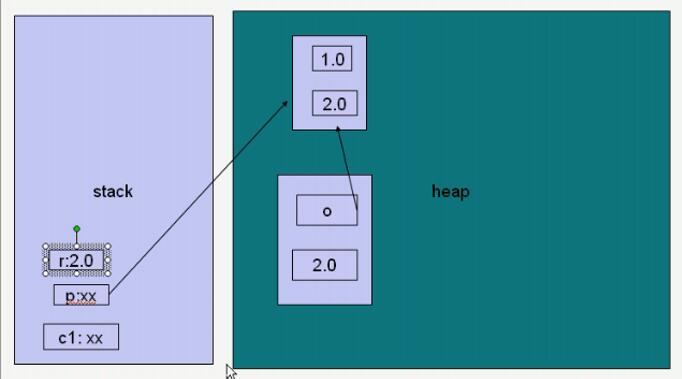**Circle c1 = new Circle(new Point(1.0,2.0), 2.0);**首先new出一个point点的对象，在堆里分配内存空间，然后该point构造方法让形式参数给point里赋值，赋值结束，然后new了一个c1，在堆内存里开辟了一块儿空间，两个参数p指向了堆里的一个引用赋值给o，r赋值给r，赋值结束后，棧里的p和r消失，形成了下图的内存调用关系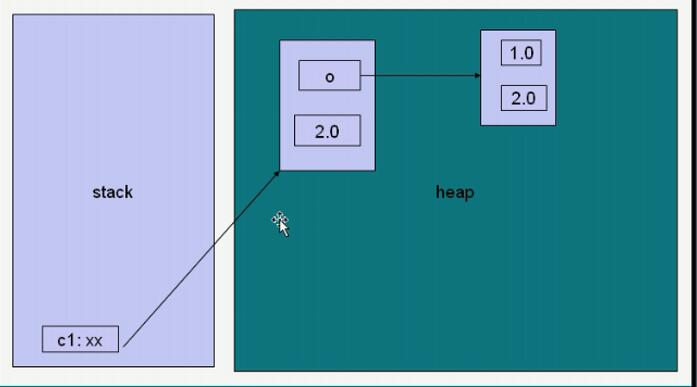System.out.println(“c1:(”+c1.getO().getX()+"," +c1.getO().getY()+"),"+c1.getR()); c1.get()调用这个方法之后，棧里两个形式参数一个指向o，而o又指向另一块儿内存，于是该形式参数直接指向该内存，从该内存取回x值后返回到棧里一块儿内存区，然后打印出来后，棧里的形参消失。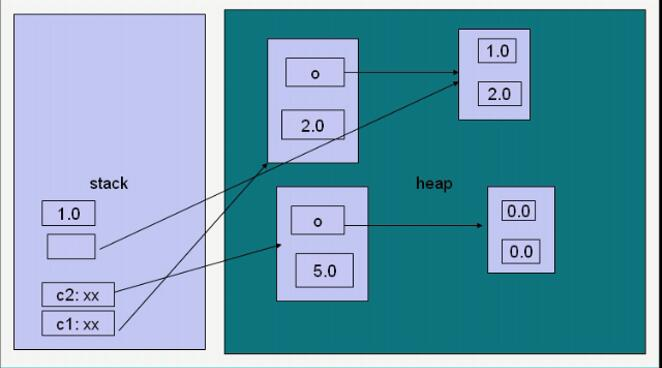c1.setO(5,6); 首先调用了seto方法，把形参x和y分别赋予了5，6的值，赋值后又把该形式参数的值调用方法setx赋予了i，i，然后，i，i把值赋给了堆内存里的参数位，调用方法完成后，棧内存里相关形参消失。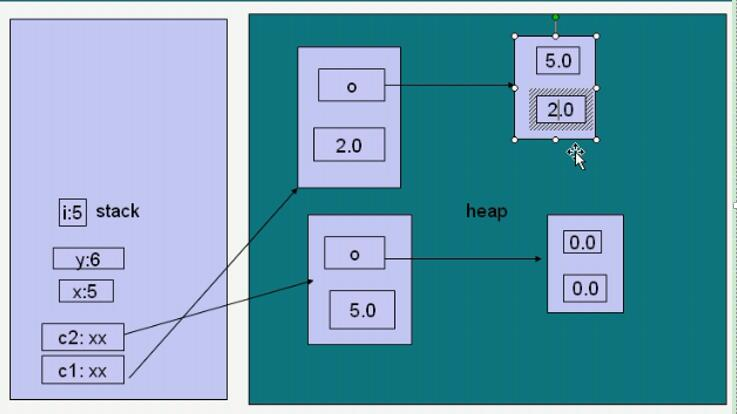Point p1 = new Point(5.2, 6.3); 首先p1在堆里new出一块儿空间来，并且赋值（棧里有两个x，y的形参，），然后c1.contains调用方法，p也指向该堆内存，然后与c1的o进行比较计算，返回一个true，打印出来以后全部消失。（p1有指向，所以不消失。）
System.out.println(c1.contains(p1));
System.out.println(c1.contains(new Point(10.0,9.0)));

##this关键字的使用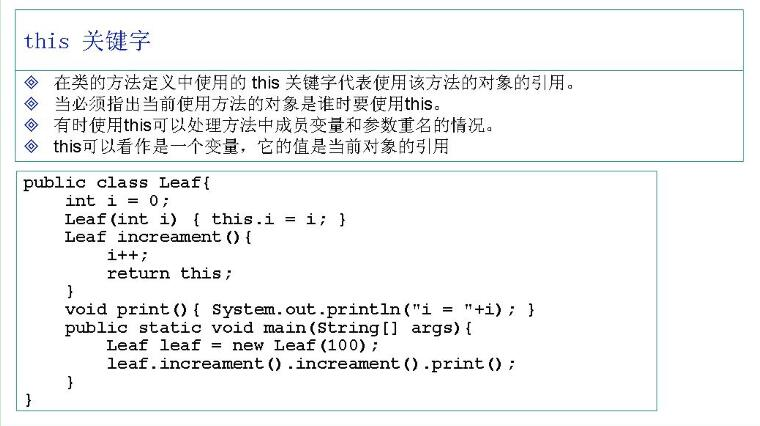this是使用该对象对自己的引用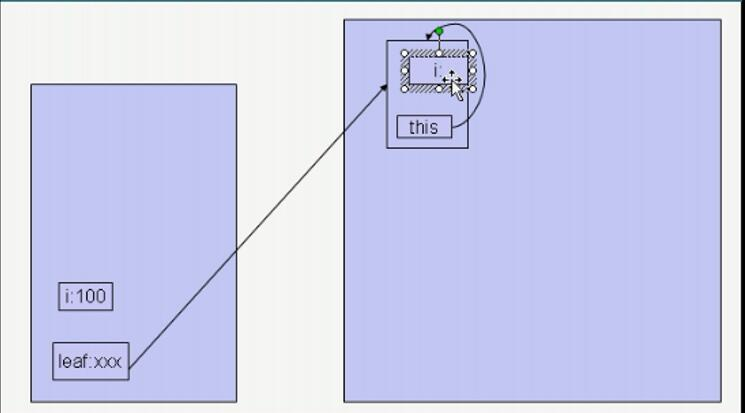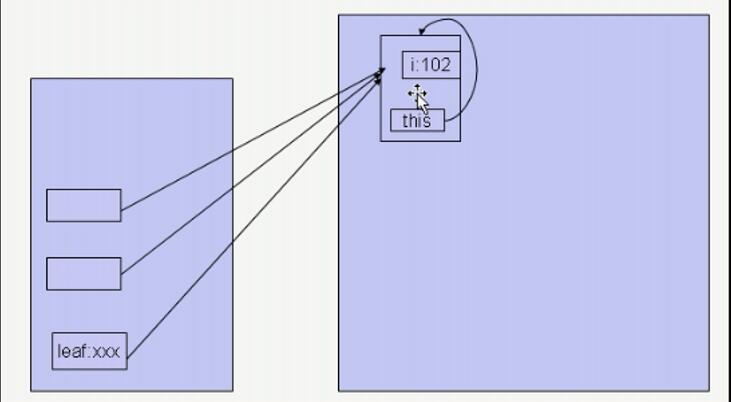##static关键字的使用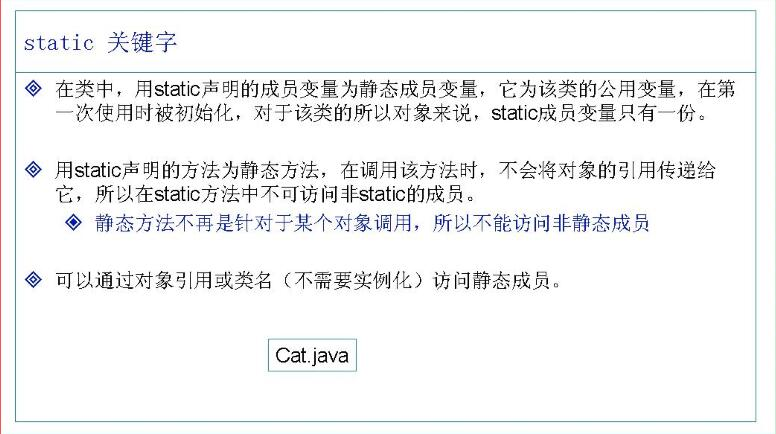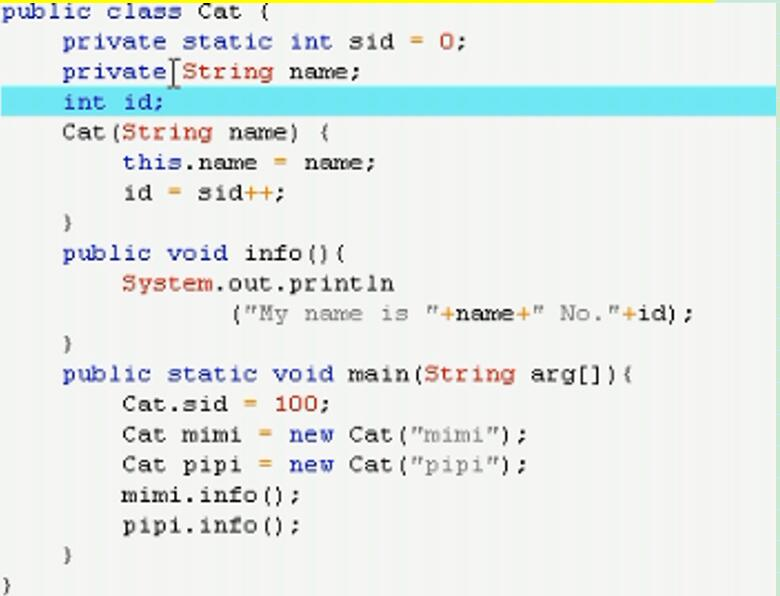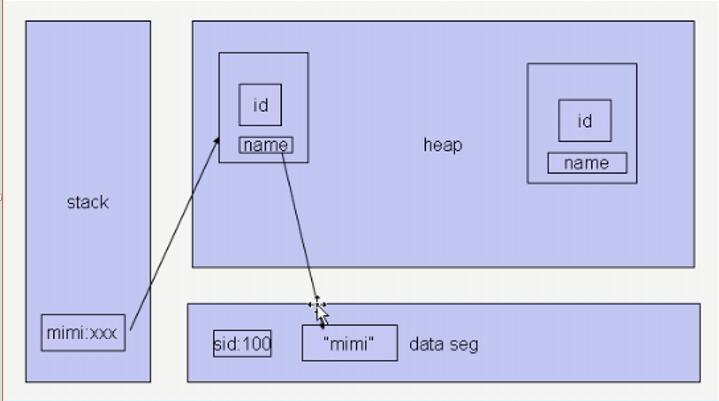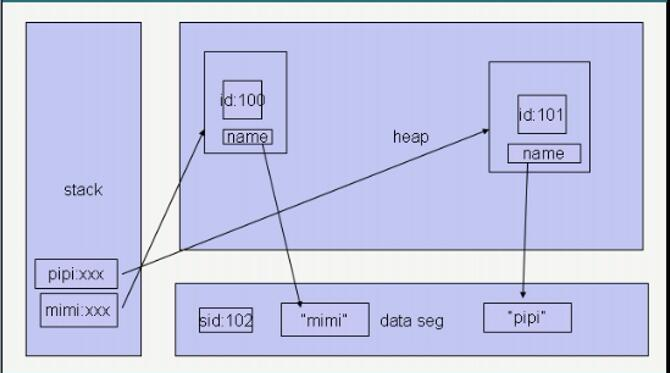##package和import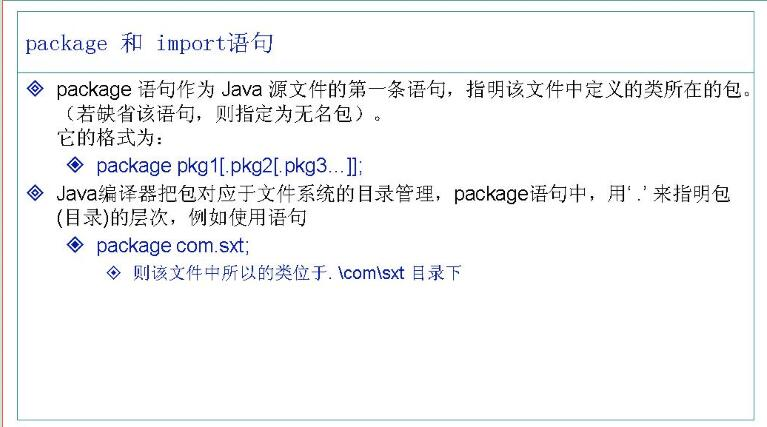**注意目录信息，一层一层往下走的**
package cn.edu.cugb.lab.Package;     //规范包名，层层设置

public class TestPackage { // 类名每个首字母都要大写

public static void main(String[] args) {
int i = TestPackage.sts(3, 4);
System.out.println(i);

}

public static int sts(int a, int b) {
if (a > b) {
return a;
} else {
return b; // 返回值都是返回到本方法的啦
}

}

}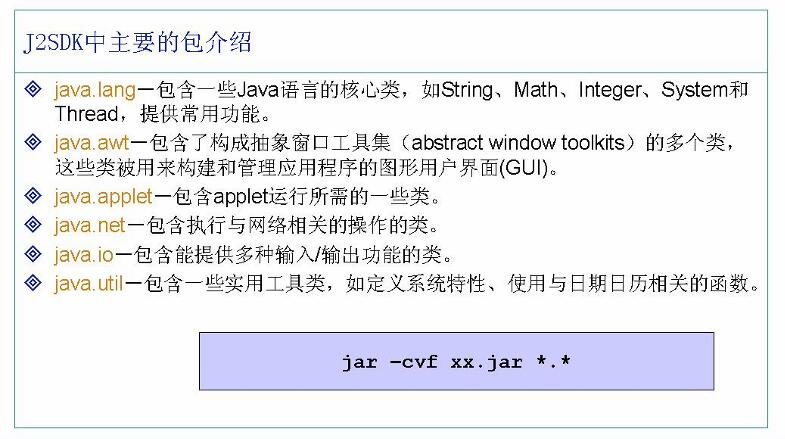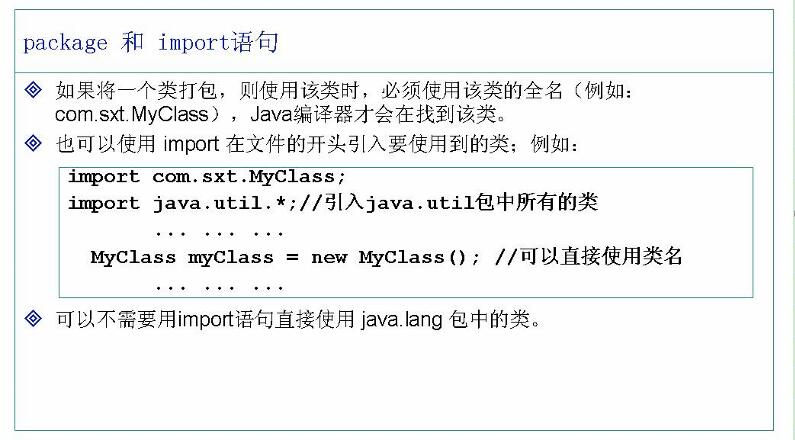*表示引入该包下所有的类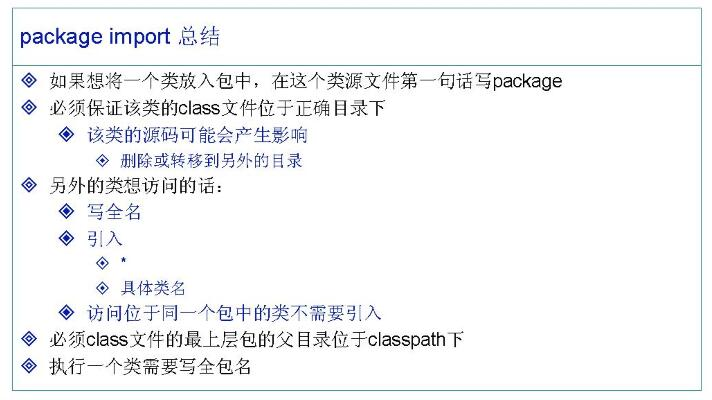Java.lang里的类不需要引用，可以直接使用，其它包的都需要引入

##访问限制符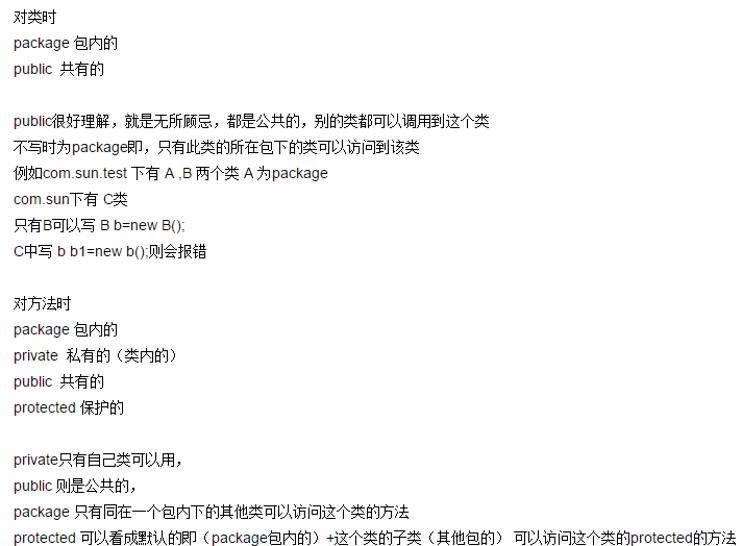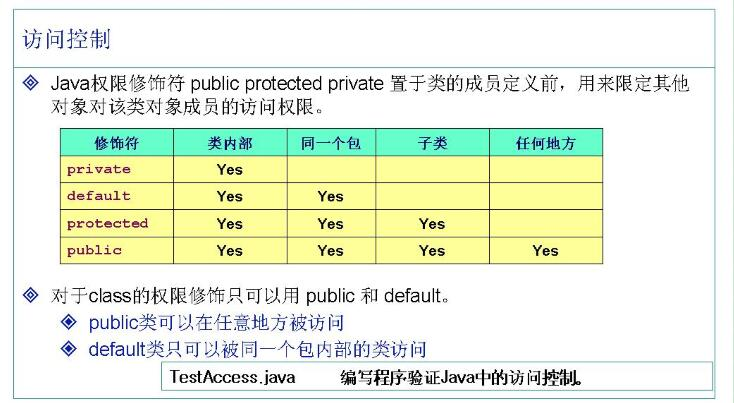Private成员变量需要访问的时候不能直接调用，需要通过get和set来赋值（公有方法里访问私有属性）。或者通过构造函数也是可以的。

#### Java基础核心技术：面向对象编程(day05-day07)

04-2707-31

#### Java基础核心技术：类特性1(day07-day09)

04-28

10-171105

#### Java基础核心技术：Java常用类(day18)

05-11

08-041353

#### Java学习笔记-------面向对象的设计思想

04-101208

#### jmu-Java-03面向对象基础-03-形状 （3 分)©️2020 CSDN 皮肤主题: 游动-白 设计师: 上身试试点击重新获取扫码支付1.余额是钱包充值的虚拟货币，按照1:1的比例进行支付金额的抵扣。
2.余额无法直接购买下载，可以购买VIP、C币套餐、付费专栏及课程。余额充值